download.file("http://www.openintro.org/stat/data/bdims.RData", destfile = "bdims.RData")
load("bdims.RData")
mdims <- subset(bdims, sex == 1)
fdims <- subset(bdims, sex == 0)

##Exercise 1. Make a histogram of men’s heights and a histogram of women’s heights. How would you compare the various aspects of the two distributions?

hist(mdims$hgt, main='male height', xlab = "height", xlim = c(140, 200), ylim = c(0, 90))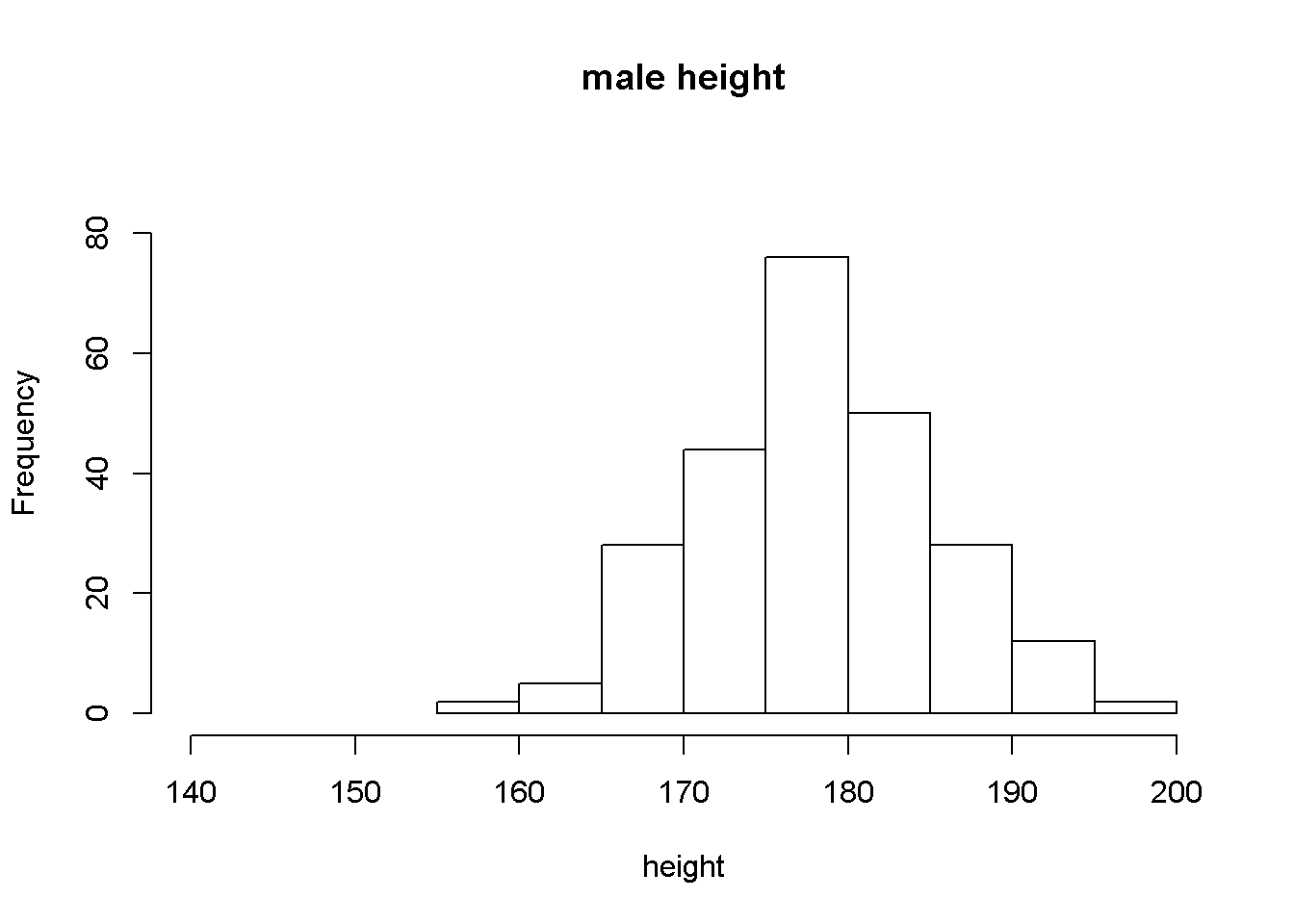hist(fdims$hgt,main = 'female height', xlab = "height", xlim = c(140, 200), ylim = c(0, 90))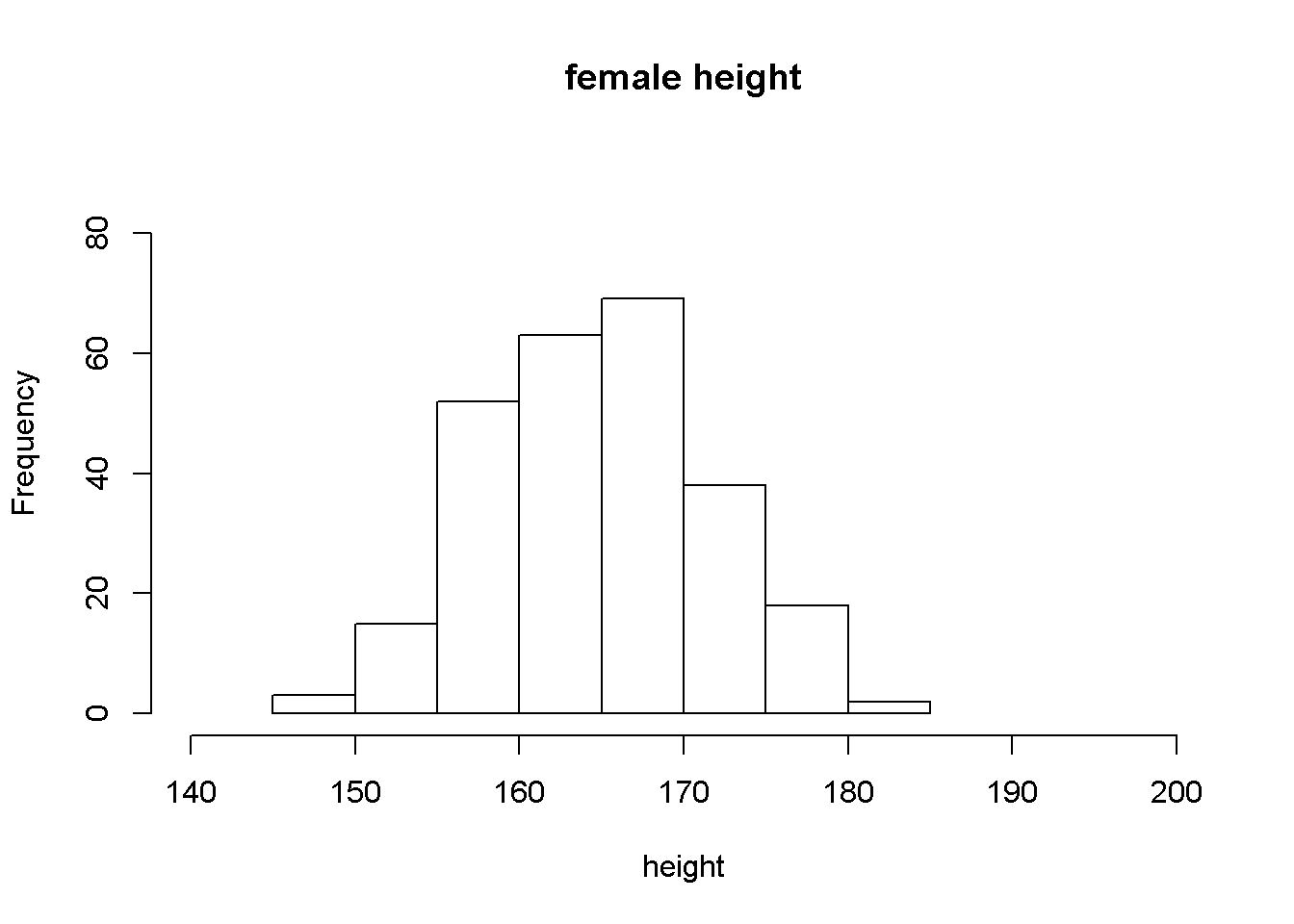###Male height seem to follow the normal distribution

fhgtmean <- mean(fdims$hgt) fhgtsd <- sd(fdims$hgt)
hist(fdims$hgt, probability = TRUE, ylim = c(0, 0.08)) x <- 140:190 y <- dnorm(x = x, mean = fhgtmean, sd = fhgtsd) lines(x = x, y = y, col = "blue")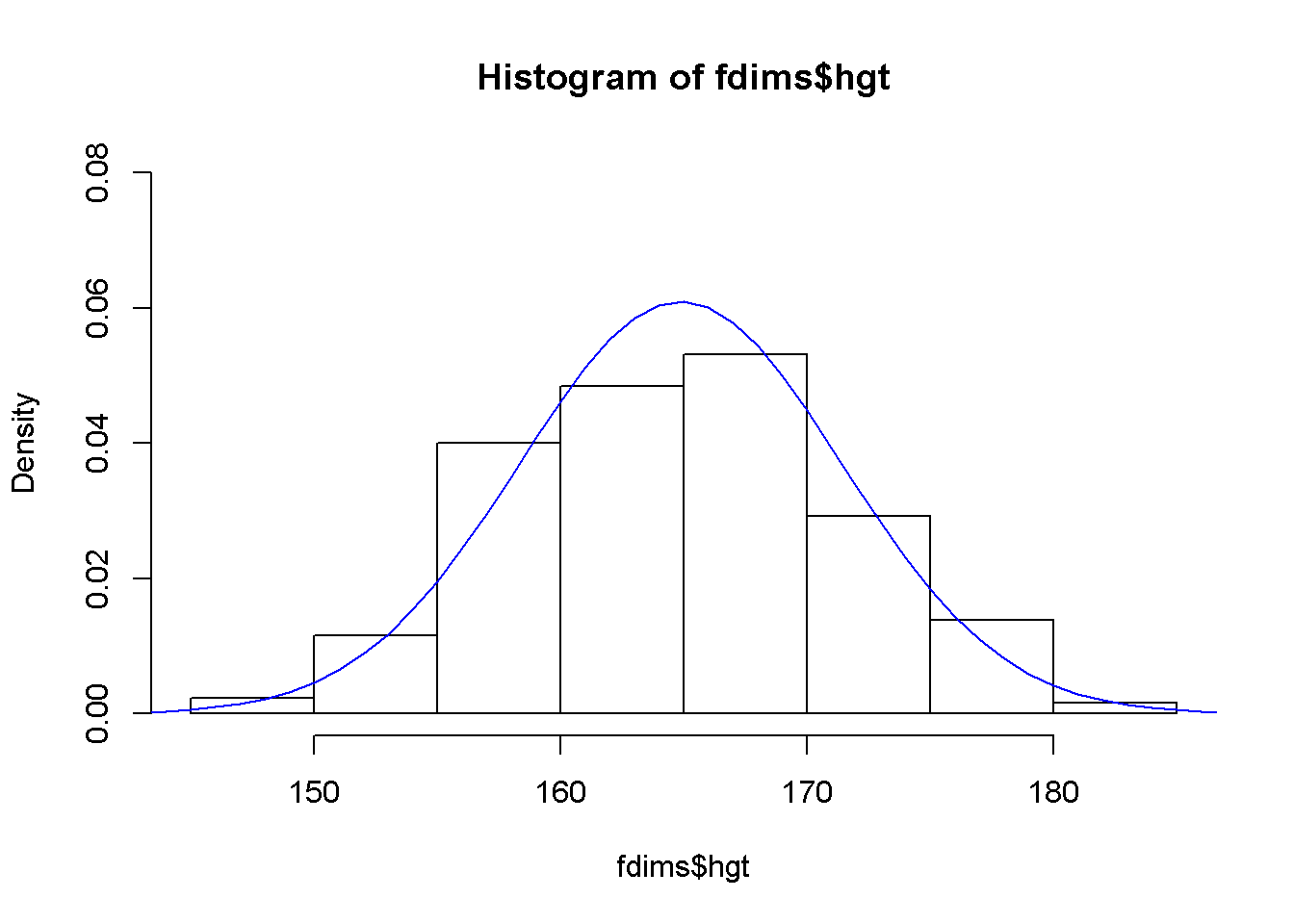##Exercise 2. Based on the this plot, does it appear that the data follow a nearly normal distribution? ### the bell shaped curve seems to suggest that the data follow a normal distribution but the data distribution from histogram does not strongly support the curve. qqnorm(fdims$hgt)
qqline(fdims$hgt)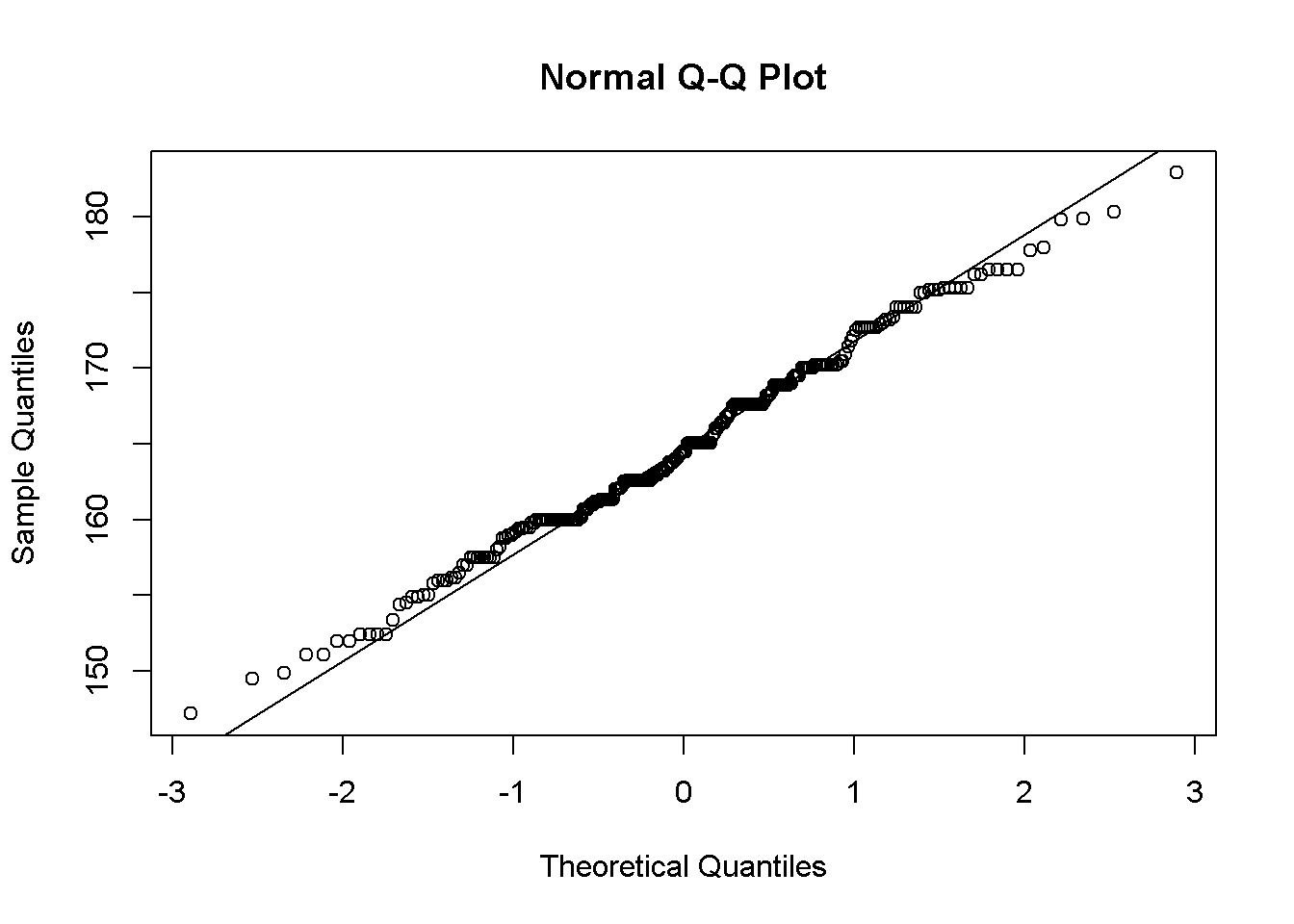sim_norm <- rnorm(n = length(fdims$hgt), mean = fhgtmean, sd = fhgtsd)

##Excercise 3. Make a normal probability plot of sim_norm. Do all of the points fall on the line? How does this plot compare to the probability plot for the real data?

qqnorm(sim_norm)
qqline(sim_norm)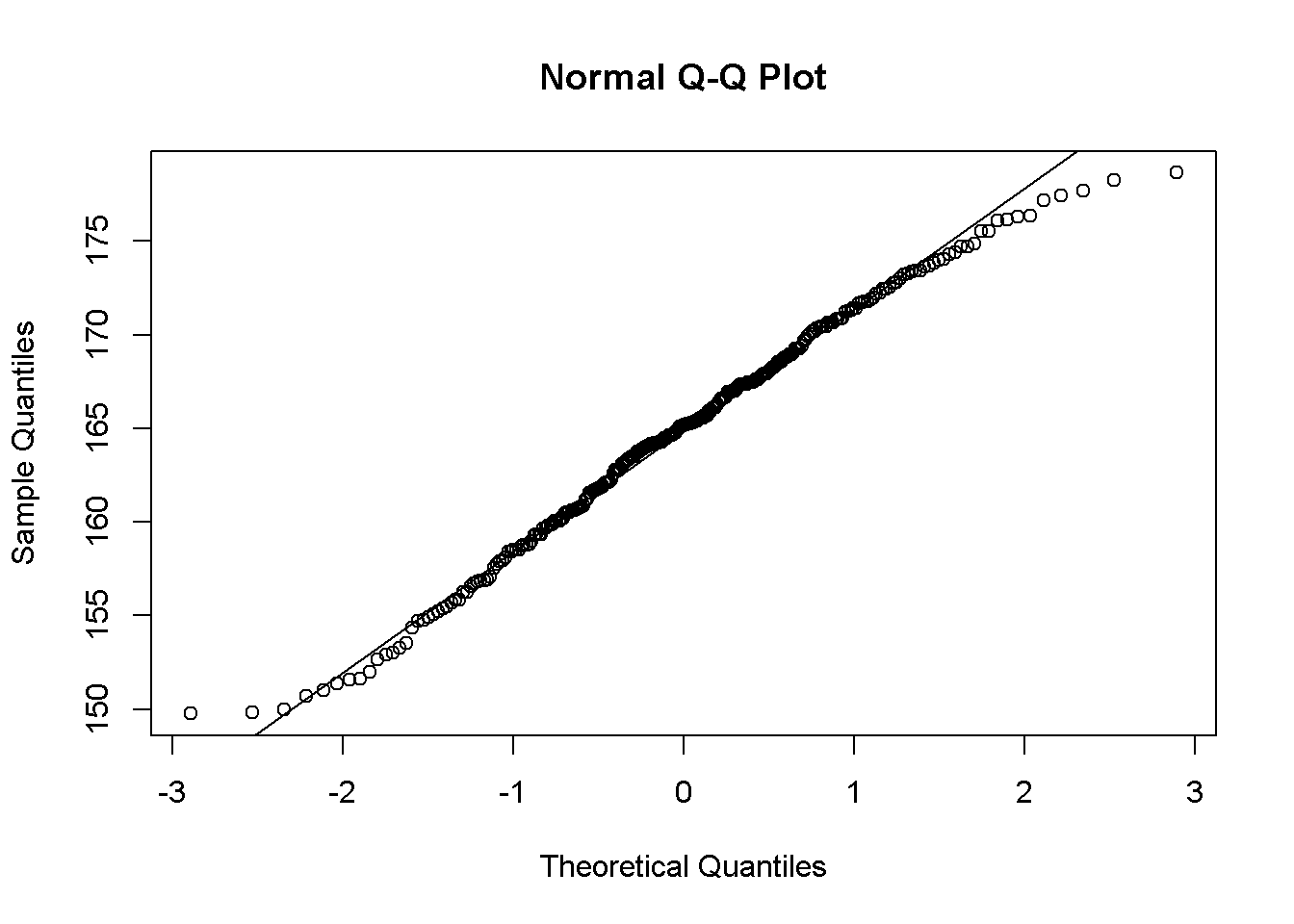###Only the middle portion of points fall on the line. The higher points between 1.5 and 3, and lower points between -3 and -2 do no fall on the line.The probability plot of real data is much more linear than that of simulated data but for both plots, majority of points do fall on the line and the ones that don’t are on the ends.

##Exercise 4. Does the normal probability plot for fdims$hgt look similar to the plots created for the simulated data? That is, do plots provide evidence that the female heights are nearly normal? qqnormsim(fdims$hgt)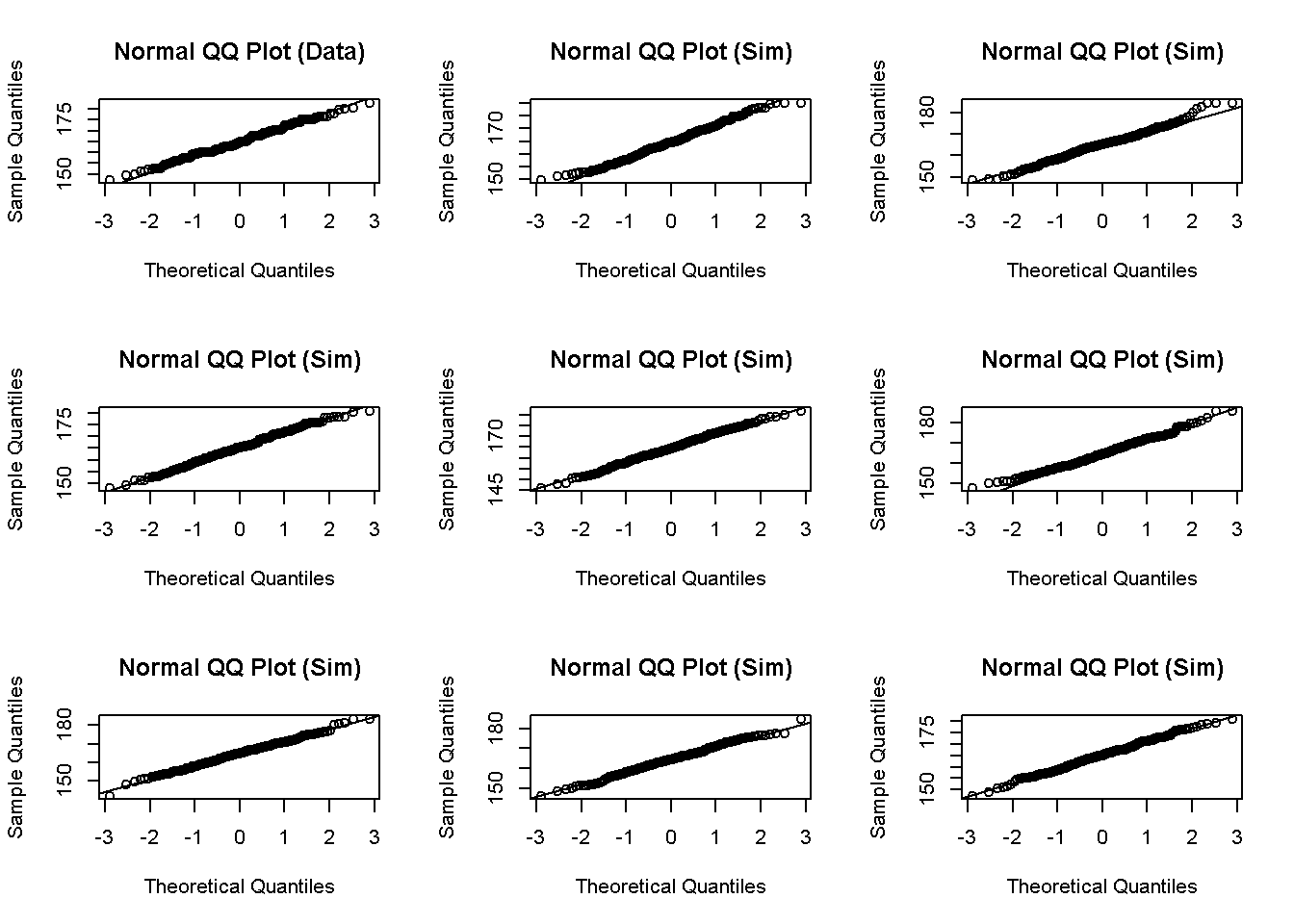###Yes, the normal probability plot for fdims$hgt look similar to the simulated probability plot. The plots do show evidence that female heights are nearly normal. ##Excercise 5. Using the same technique, determine whether or not female weights appear to come from a normal distribution. qqnormsim(fdims$wgt)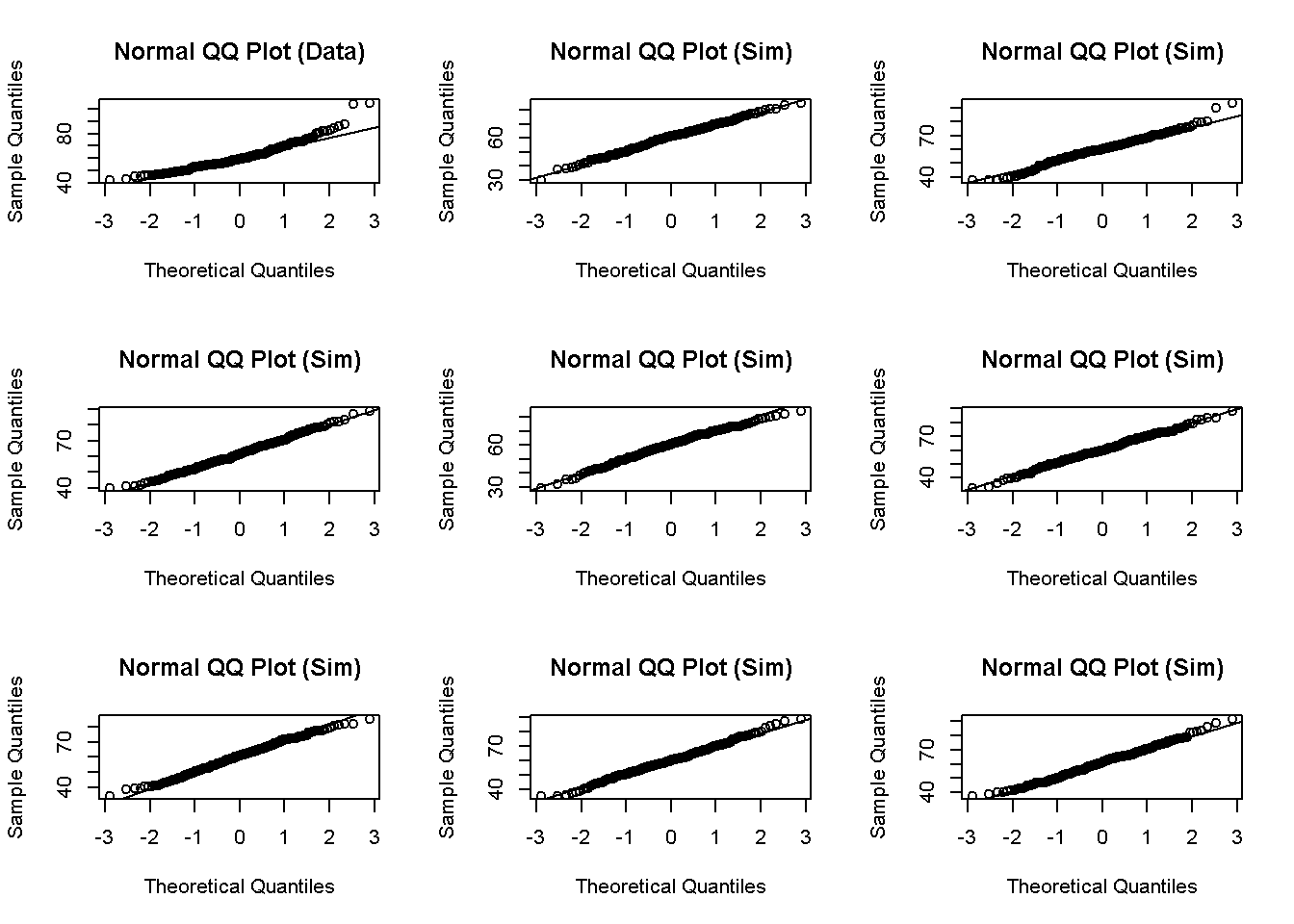###the female weights plot seems to follow the normal distribution but QQ data plot shows evidence of data being right skewed.

1 - pnorm(q = 182, mean = fhgtmean, sd = fhgtsd)
##  0.004434387
sum(fdims$hgt > 182) / length(fdims$hgt)
##  0.003846154

##Exercise 6. Write out two probability questions that you would like to answer; one regarding female heights and one regarding female weights. Calculate the those probabilities using both the theoretical normal distribution as well as the empirical distribution (four probabilities in all). Which variable, height or weight, had a closer agreement between the two methods?

wtmean=mean(fdims$wgt) wtsd=sd(fdims$wgt)

###What percent of females have a height of 170cm or more?

1-pnorm(170, mean = fhgtmean, sd=fhgtsd)
##  0.2166669
sum(fdims$hgt >170)/length(fdims$hgt)
##  0.2230769

###What percent of females have a height of 80kg or more?

1-pnorm(q=80, mean = wtmean, sd = wtsd)
##  0.02182199
sum(fdims$wgt >80)/length(fdims$wgt)
##  0.04230769

###Height was the variable that had a closer agreement between the two different methods.

###What percent of females have a weight of 70kg or more?

#On your own ##Now let’s consider some of the other variables in the body dimensions data set. Using the figures at the end of the exercises, match the histogram to its normal probability plot. All of the variables have been standardized (first subtract the mean, then divide by the standard deviation), so the units won’t be of any help. If you are uncertain based on these figures, generate the plots in R to check.

###a. The histogram for female biiliac (pelvic) diameter (bii.di) belongs to normal probability plot letter B.

qqnorm(fdims$bii.di) qqline(fdims$bii.di)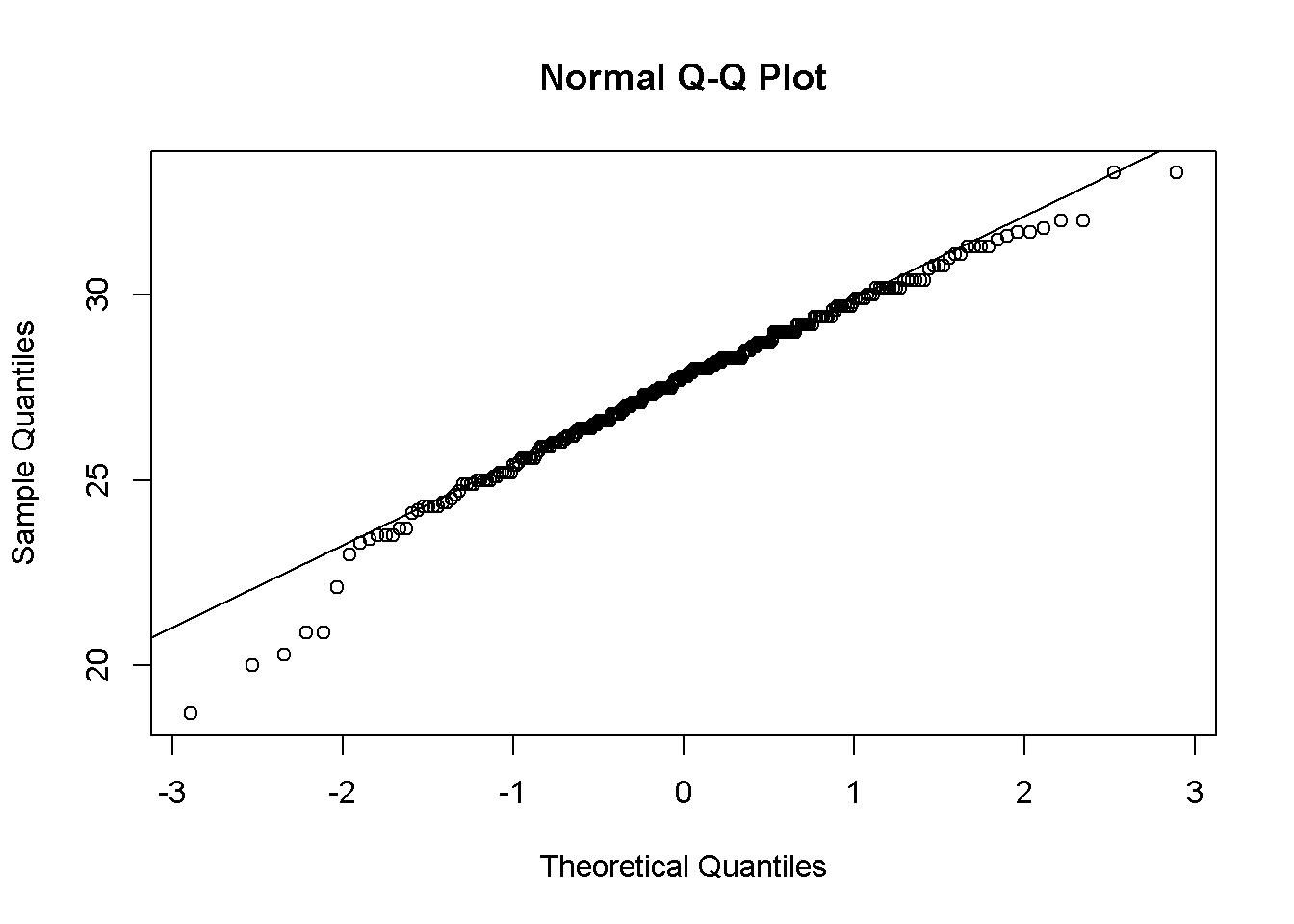###b. The histogram for female elbow diameter (elb.di) belongs to normal probability plot letter C.

qqnorm(fdims$elb.di) qqline(fdims$elb.di)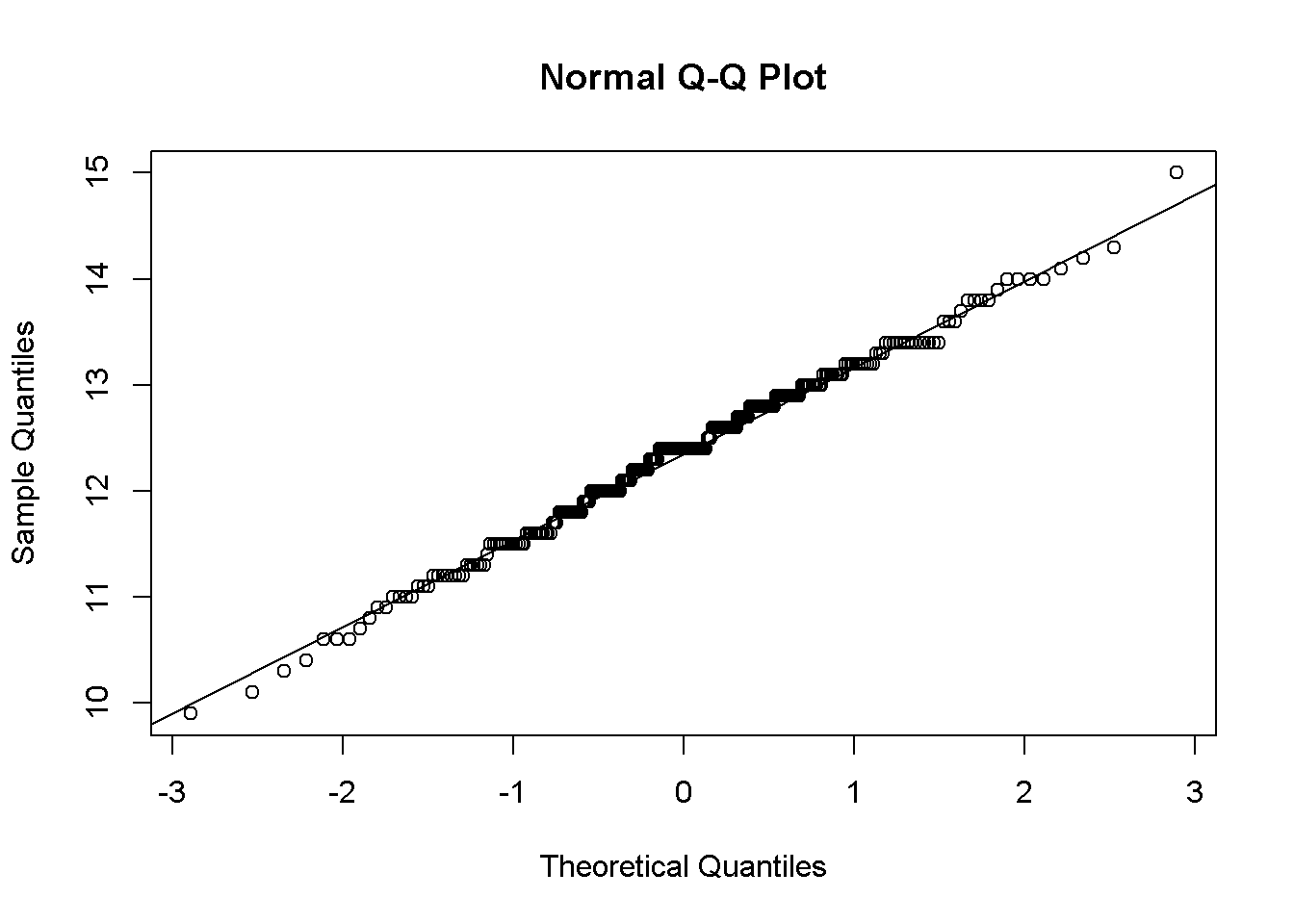###c. The histogram for general age (age) belongs to normal probability plot letter D.

qqnorm(fdims$age) qqline(fdims$age)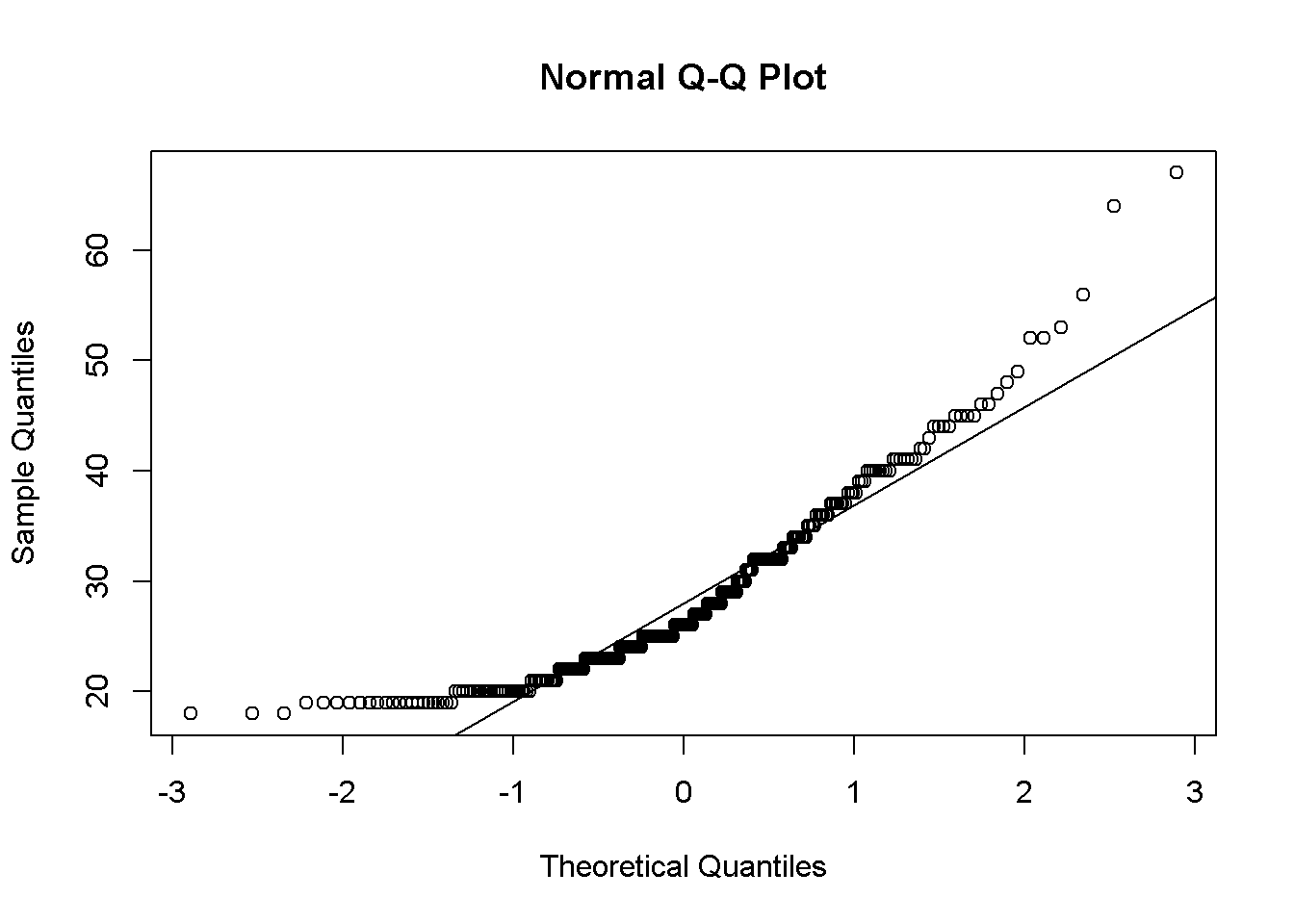###d. The histogram for female chest depth (che.de) belongs to normal probability plot letter A.

qqnorm(fdims$che.de) qqline(fdims$che.de)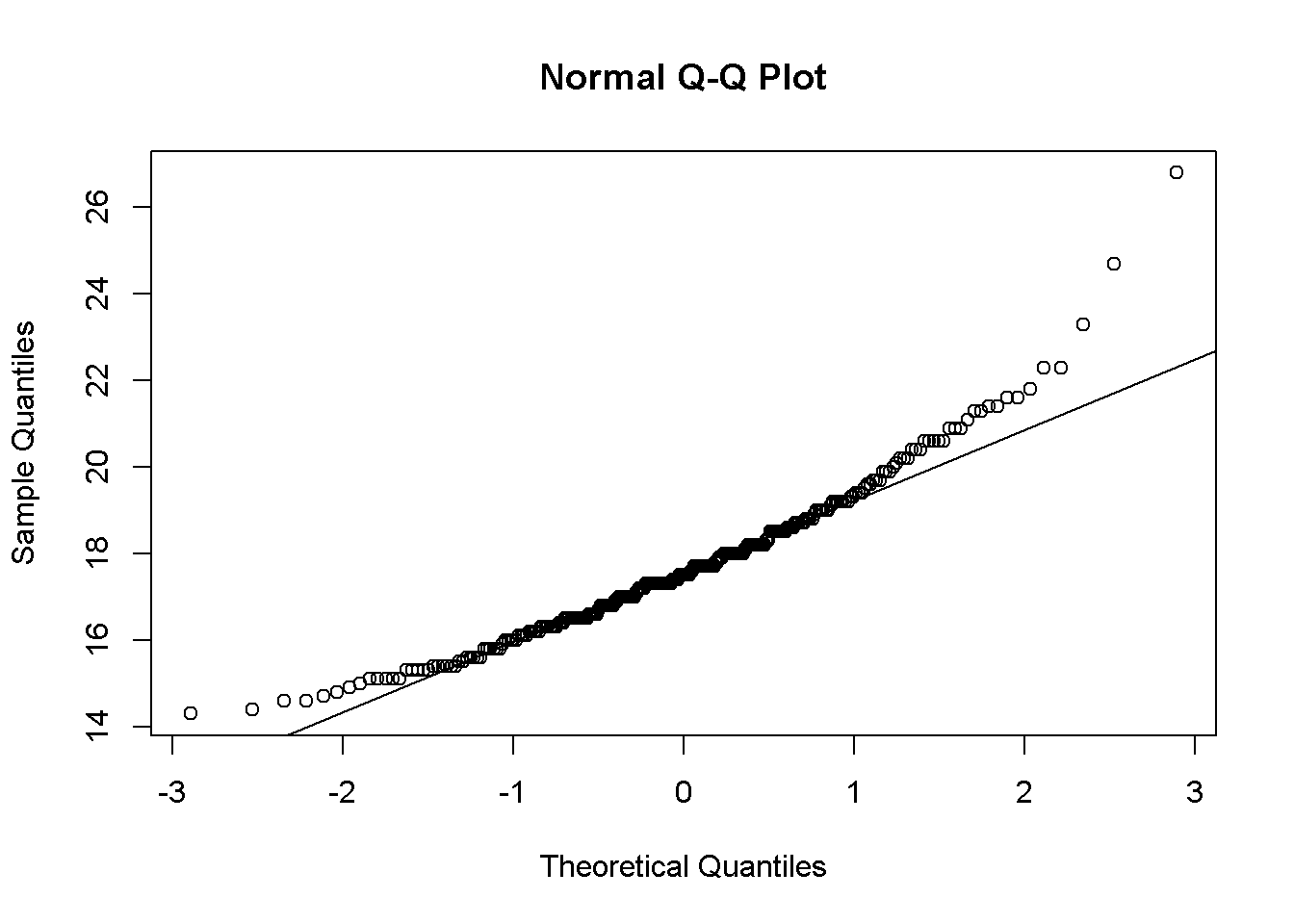##Note that normal probability plots C and D have a slight stepwise pattern.Why do you think this is the case? ###This is likely due to discrete scale because age and chest depth are reported as an integer whereas elbow and pelvic can be reported in decimal places.

##As you can see, normal probability plots can be used both to assess normality and visualize skewness. Make a normal probability plot for female knee diameter (kne.di). Based on this normal probability plot, is this variable left skewed, symmetric, or right skewed? Use a histogram to confirm your findings.

qqnorm(fdims$kne.di) qqline(fdims$kne.di)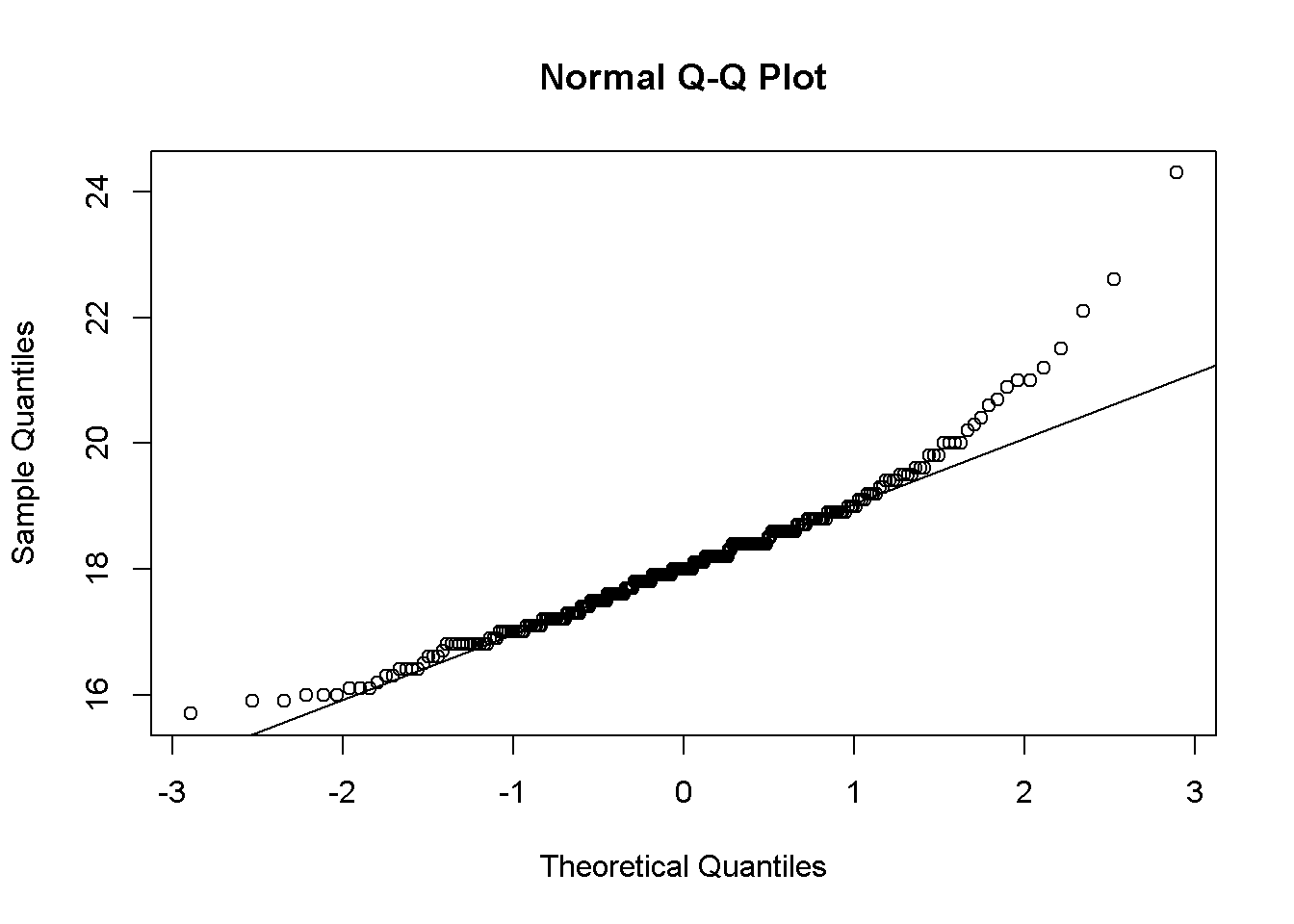###The probability plot indicated that the the female knee diameter distribution to be right skewed.